Warning: Illegal string offset 'html' in /home/hsn/public_html/forum/cache/skin_cache/cacheid_1/skin_topic.php on line 909

HSN Maths Quiz - HSN forum - Page 2# HSN Maths Quiz

23 replies to this topic

• Gender:Male

Posted 11 January 2006 - 09:17 AM

Q1

3 - 6x - x^2
= -x^2 – 6x + 3
= -[x^2 + 6x – 3]
= -[(x^2 + 6x) - 3]
= -[(x + 3)^2 – 3 – 9]
= -(x + 3)^2 + 12

Q4

b^2 – 4ac = 0
(-2p – 6)^2 – (4 * (p + 1) * 3p) = 0
(4p^2 + 24p + 36) – (12p^2 + 12p) = 0
-8p^2 + 12p + 36 = 0
2p^2 – 3p – 9 = 0
p = -2/3 and p = 3

### #22George

Child Prodigy

••• 720 posts
• Location:West Lothian
• Gender:Male

Posted 11 January 2006 - 04:23 PM

QUOTE(duncad @ Jan 11 2006, 06:29 AM)

The maths needs to be input in 'latex' code.

This needs to go between the  tags, if you have maths in a sentence, or [display] [/display] if you want to do a few lines of maths.

Here's an example: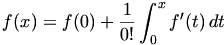The code for this is:
CODE
[display]f(x) = f(0) + \frac{1}{0!}\int_0^x{f'(t) \, dt}[/display]

I'll just demonstrate a few common commands:

x^n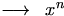x_n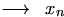\frac{a}{b}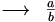\int_{a}^{b} {f(x) \, dx}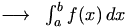\piTo line up equations at the = sign, use &= like this:
CODE
[display]
y &= (x-1)(x+3)  \\
&= x^2 + 3x - x - 3  \\
&= x^2 +2x -3
[/display]

Gives: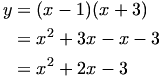Also, notice that \\ takes a new line in [display] mode.

For more details, there are lots of good tutorials on the web, like this one### #23Kara

• Gender:Female

Posted 04 May 2006 - 04:43 PM

Q1 - should it not be :

f'(x)= 1/2(8x-5) ^-1/2 times 8

= 4(8x-5)^-1/2
= 4/the sqaure root of (8x - 5)

If not where am i going wrong?

### #24Dave

Ruler (but not owner) of hsn

•• Moderators
•• 4,252 posts
• Location:kilmarnock(ok kilmaurs)
• Interests:programming, exercising, brass band, using this board
• Gender:Male

Posted 04 May 2006 - 05:23 PM

yes it is

If i am not here i am somewhere else

#### 1 user(s) are reading this topic

0 members, 1 guests, 0 anonymous users